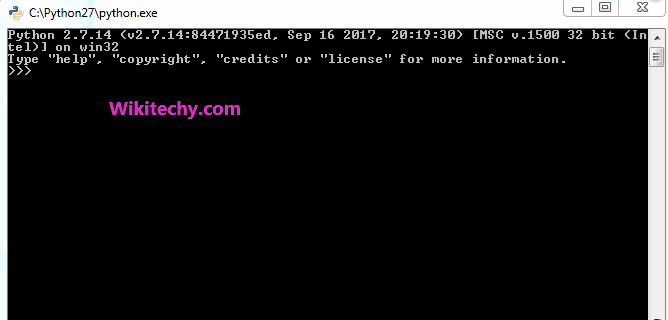# python tutorial - Python Float Precision | Precision Handling in Python - learn python - python programmingLearn Python - Python tutorial - python float precision - Python examples - Python programs

Python in its definition allows to handle precision of floating point numbers in several ways using different functions. Most of them are defined under the “math” module.

1. trunc() :- This function is used to eliminate all decimal part of the floating point number and return the integer without the decimal part.

2. ceil() :- This function is used to print the least integer greater than the given number.

3. floor() :- This function is used to print the greatest integer smaller than the given integer.

python - Sample - python code :

python tutorial - Output :

```The integral value of number is : 3
The smallest integer greater than number is : 4
The greatest integer smaller than number is : 3
```

Setting Precision

There are many ways to set precision of floating point value. Some of them is discussed below.

1. Using “%” :- “%” operator is used to format as well as set precision in python. This is similar to “printf” statement in C programming.

2. Using format() :- This is yet another way to format the string for setting precision.

3. Using round(x,n) :- This function takes 2 arguments, number and the number till which we want decimal part rounded.

python - Sample - python code :

python tutorial - Output :

```The value of number till 2 decimal place(using %) is : 3.45
The value of number till 2 decimal place(using format()) is : 3.45
The value of number till 2 decimal place(using round()) is : 3.45
```

Wikitechy tutorial site provides you all the learn python , learn python programming , study python programming , python lessons online , python programming for beginner